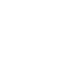Home MS Office Guide

# How To Implement The SUM Function To Find The Sum of Numbers In A Row Or Column in MS Excel?

Microsoft Excel is an extremely popular Office application among students, offices, researchers. It facilitates a plethora of options to create a huge table. You can create graphs, pie charts, trends of a particular business or scientific model and so on. The number of features are almost endless.

Microsoft Office Excel is humongously used in research and study purposes. It has an amazing set of features that help users to perform complex mathematical formulae and functions. The process of creating formulas, or checking them for reference is extremely easy in case of MS Excel.

There are many built-in functions that help you perform arithmetic, trigonometric and logical operations. One commonly used function is the SUM function. This function is used to find the addition of numbers in a row or in a column. The process is very simple and may be known to many. This article is for beginners:

## Steps To Implement The SUM Function To Find The Sum of Numbers In A Row Or Column in MS Excel

1.In a table, first select an empty cell, where you want to get the sum total of the numbers.

2. Next, type in the cell = [Remember, while you are implementing any function, it is a convention that you put the ‘=’ equals to sign in the beginning]. Otherwise, you won’t be able to accomplish your operation.

3. Next, type the SUM function

4. Next, type an opening paranthesis. (

5. Now select the row or column range, or simply type the range. The range convention is something like this: first column/row index : end column or row index. If you select all the required columns, then also, it will appear like this. So, the format will look like the one shown in the image.

6. Finally hit Enter to get the sum total of the rows or columns.